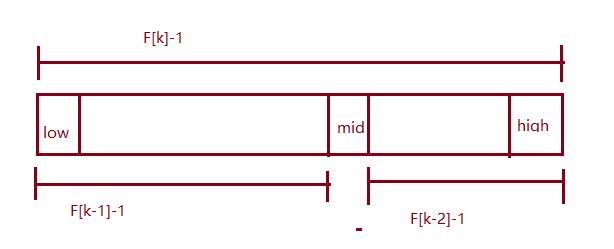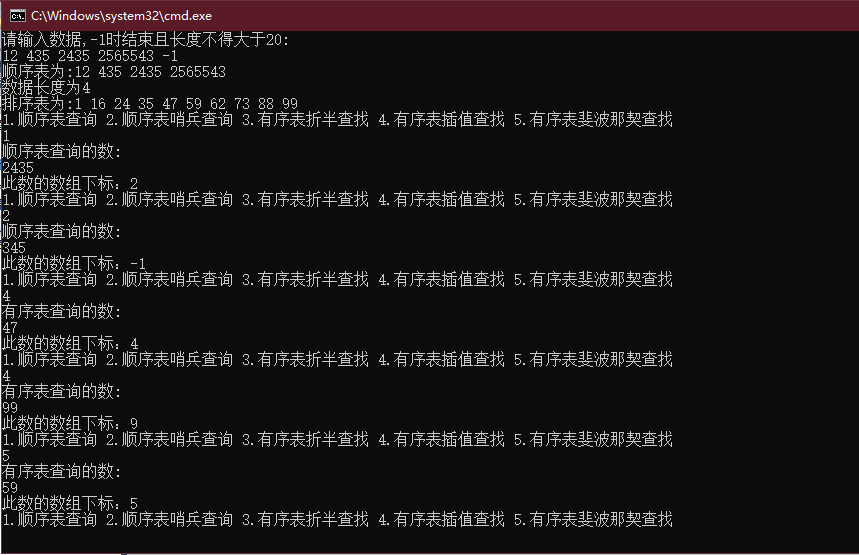• 顺序表查找(Sequential Search)又叫线性表查找，是最基本的查找法，从第一个开始到最后一个逐个与关键字比较。成功不成功都返回。 //顺序表查找 int SequentialSearch(int* a,int n, int key) { for(int i=0;i;i++)...
顺序表查找(Sequential Search)又叫线性表查找，是最基本的查找法，从第一个开始到最后一个逐个与关键字比较。成功不成功都返回。

//顺序表查找
int SequentialSearch(int* a,int n, int key)
{
for(int i=0;i<n;i++)
{
if(key==a[i])
{
return i;
}
}
else
return -1;
}上面代码缺点是每次取i都要跟n 比较；//顺序表查找优化
int SequentialSearch2(int* a,int n, int key)
{
a[n]=key;
int i=0;
while(key != a[i])
{
i++;
}
return i;//返回n表示失败了
}此时代码从尾部开始查找，这种查找避免了每次查找i跟n的比较 时间复杂度为O(n);

展开全文• 顺序表查找有序表查找 对顺序表进行二分查找 索引顺序查找表 索引顺序查找表也就是分块查找 把线性表分为若干块，每一块的关键字都小于下一块的最小关键字。 索引按顺序排列进行二分查找，在对找到的块进行顺序查找
顺序表查找

有序表查找

对顺序表进行二分查找
索引顺序查找表

索引顺序查找表也就是分块查找
把线性表分为若干块，每一块的关键字都小于下一块的最小关键字。
索引按顺序排列进行二分查找，在对找到的块进行顺序查找

展开全文• 顺序表查找，逐一比较，它有以下算法 1.顺序查找 2.顺序查找优化（加了个哨兵） 代码： /* * 顺序查找不等同与顺序表就已经是有序的了，这里需要注意 * 时间复杂度为0(n) * 空间复杂度为1 */ public ...
顺序表查找，逐一比较，它有以下算法

1.顺序查找

2.顺序查找优化（加了个哨兵）

代码：

/*
* 顺序查找不等同与顺序表就已经是有序的了，这里需要注意
* 时间复杂度为0(n)
* 空间复杂度为1
*/
public class SequentialSearch {

/*
* 顺序表查找算法
* @param a 数组
* @param n 数组大小
* @param key 要查找的关键字
* @return 返回查找的位置
*/
public int sequentialSearch1(int[] a, int n, int key) {
for(int i = 0;i < n;i++) {
if(a[i] == key) {
return i;
}
}
return 0;

}

/*
* 顺序表查找优化-有哨兵顺序查找
* 优化方向：设置一个哨兵解决不需要每次让i与n做比较
* 即不需要每次循环都判断i是否越界
*
* @param a 数组
* @param n 数组大小
* @param key 要查找的关键字
* @return 返回查找的位置
*/
public int sequentialSearch2(int[] a, int n, int key) {
//设置a为关键字值，也就是哨兵
a = key;
//从后往前查找
int i = n;
while(a[i] != key) {
i--;
}
//返回0说明查找失败
return i;
}
}


展开全文• 顺序表查找：顺序查找，哨兵查找 有序表查找：折半查找，插值查找，斐波那契查找
静态查找前言顺序表查找顺序查找哨兵查找有序表查找折半查找插值查找斐波那契查找真正实现代码功能运行结果后记
前言
搜索引擎就是利用了查找的技术，查找方式按照操作方式有两大种，分别是静态查找和动态查找。静态查找只做查找，动态查找在查找过程中插入不存在的元素，删除已存在的元素。
本篇只讨论有关静态查找的内容
找不到返回-1
顺序表查找
顺序表查找分为顺序查找和优化的哨兵查找。
顺序查找
int Sequential_Search(int *a,int n,int key)
{
int i;
for(i=0;i<n;i++)
{
if(a[i]==key)
return i;
}
return -1;

}

哨兵查找
在优化的查找中，设置了一个哨兵，免去了查找过程中每次比较后都要判断查找位置是否越界。总数据较多时，效率提高很大
int Sequential_Search1(int *a,int n,int key)
{
int i;
a[-1] = key;//设置哨兵
i = n;
while(a[i]!=key)
{
i--;
}
return i;//返回-1则说明查找失败
}

有序表查找
有序表就是有排列顺序的表
折半查找适用于数据量较大的
插值查找适用于表长比较大，关键字分布广的
斐波那契适用于数据分布极端不均匀的
折半查找
int Binary_Search(int *a,int n,int key)
{
int low,high,mid;
low = 0;//定义最低下标为记录首位
high = 9;//定义最高下标为记录末尾
while(low<=high)
{
mid = (low+high)/2;//折半
if(key<a[mid])
high = mid-1;
else if(key>a[mid])
low = mid+1;
else
return mid;
}
return -1;
}

插值查找
插值查找的核心在于插值的计算公式。查找关键字key与查找表中最大最小记录的关键字比较后的查找方法。
代码就是折半查找中注释折半那一行替换为查找这一行就行了
mid = low+(high-low)*(key-a[low])/(a[high]-a[low]);//插值

斐波那契查找
斐波那契查找利用了黄金分割率原理实现
查找的核心在于：
当key = a[mid]时，查找成功；
当key < a[mid]时，新范围是第low到mid-1个，此时范围个数为F[k-1]-1个；
当key 》 a[mid]时，新范围是第mid+1到high个，此时范围个数为F[k-2]-1个；int Fibonacci_Search(int *a,int n,int key)
{

int F;
F = 0;
F = 1;
for(int m = 2;m<30;m++)
F[m] = F[m-1]+F[m-2];//斐波那契数组

int low,high,mid,i,k;
low = 0;//定义最低下标为记录首位
high = n;//定义最高下标为记录末尾
k = 0;
while(n>F[k]-1)//记录n位于斐波那契数列的位置
k++;
for(i=n;i<F[k]-1;i++)//将不满的数值补全
a[i] = a[n];
while(low<=high)
{
mid = low+F[k-1]-1;//计算当前分隔的下标
if(key<a[mid])
{
high = mid-1;//最高下标调整到mid-1处
k = k-1;//斐波那契下标减一位
}
else if(key>a[mid])//若查找记录大于分隔记录
{
low = mid+1;//最低下标调整到mid+1处
k = k-2;//斐波那契下标减一二位
}
else
{
if(mid<=n)
return mid;
else
return n;//若mid=n，说明是补全数值，返回n
}
}
return -1;
}
}

真正实现代码功能
花了较长时间把这些代码的功能实现了。
注意创建一个比较自由的数组，我们可以用顺序表的结构体来表示数组，但是还有一种方法，与结构体表示有异曲同工之妙，首先创建一个数组，然后往其中填充数据，再用一个子函数求出数据长度。
顺序表数据是自己填充
有序表是系统自带，长度固定为9
#include<stdio.h>
#include<stdlib.h>

#define M 20

void Init(int *a);//初始化数组
void Display(int *a);//显示数组
int number(int *a);//求数据长度
int Sequential_Search(int *a,int n,int key);//顺序查找
int Sequential_Search1(int *a,int n,int key);//顺序哨兵查找
int Binary_Search(int *a,int n,int key);//有序表折半查找
int Interpolation_Search(int *a,int n,int key);//有序表插值查找
int Fibonacci_Search(int *a,int n,int key);//有序表斐波那契查找

int main()
{
int i,x,n;
int len;
int a;
Init(a);
printf("顺序表为:");
Display(a);
len = number(a);
printf("数据长度为%d\n",len);
int b = {1,16,24,35,47,59,62,73,88,99};
printf("排序表为:");
Display(b);
while(1)
{
printf("1.顺序表查询 2.顺序表哨兵查询 3.有序表折半查找 4.有序表插值查找 5.有序表斐波那契查找\n");
scanf("%d",&x);
switch(x)
{
case 1:
printf("顺序表查询的数:\n");
scanf("%d",&n);
i = Sequential_Search(a,len,n);
printf("此数的数组下标：%d\n",i);
break;
case 2:
printf("顺序表查询的数:\n");
scanf("%d",&n);
i = Sequential_Search1(a,len,n);
printf("此数的数组下标：%d\n",i);
break;
case 3:
printf("有序表查询的数:\n");
scanf("%d",&n);
i = Binary_Search(b,9,n);
printf("此数的数组下标：%d\n",i);
break;
case 4:
printf("有序表查询的数:\n");
scanf("%d",&n);
i = Interpolation_Search(b,9,n);
printf("此数的数组下标：%d\n",i);
break;
case 5:
printf("有序表查询的数:\n");
scanf("%d",&n);
i = Fibonacci_Search(b,9,n);
printf("此数的数组下标：%d\n",i);
break;
}
}
}

void Init(int *a)
{
int i = 0;
int x = 0;
printf("请输入数据,-1时结束且长度不得大于20:\n");
while(x!=-1)
{
scanf("%d",&x);
if(x!=-1)
{
a[i] = x;
i++;
}
}
a[i] = '\0';
}

void Display(int *a)
{
for(int i=0;a[i]!='\0';i++)
{
printf("%d ",a[i]);
}
printf("\n");
}

int number(int *a)
{
int i;
for(i =0;a[i]!='\0';)
{
i++;
}
return i;
}

int Sequential_Search(int *a,int n,int key)
{
int i;
for(i=0;i<n;i++)
{
if(a[i]==key)
return i;
}
return -1;

}

int Sequential_Search1(int *a,int n,int key)
{
int i;
a[-1] = key;//设置哨兵
i = n;
while(a[i]!=key)
{
i--;
}
return i;//返回-1则说明查找失败
}

int Binary_Search(int *a,int n,int key)
{
int low,high,mid;
low = 0;//定义最低下标为记录首位
high = 9;//定义最高下标为记录末尾
while(low<=high)
{
mid = (low+high)/2;//折半
if(key<a[mid])
high = mid-1;
else if(key>a[mid])
low = mid+1;
else
return mid;
}
return -1;
}

int Interpolation_Search(int *a,int n,int key)
{
int low,high,mid;
low = 0;
high = 9;
while(low<=high)
{
mid = low+(high-low)*(key-a[low])/(a[high]-a[low]);//插值
if(key<a[mid])
high = mid-1;
else if(key>a[mid])
low = mid+1;
else
return mid;
}
return -1;
}

int Fibonacci_Search(int *a,int n,int key)
{

int F;
F = 0;
F = 1;
for(int m = 2;m<30;m++)
F[m] = F[m-1]+F[m-2];//斐波那契数组

int low,high,mid,i,k;
low = 0;//定义最低下标为记录首位
high = n;//定义最高下标为记录末尾
k = 0;
while(n>F[k]-1)//记录n位于斐波那契数列的位置
k++;
for(i=n;i<F[k]-1;i++)//将不满的数值补全
a[i] = a[n];
while(low<=high)
{
mid = low+F[k-1]-1;//计算当前分隔的下标
if(key<a[mid])
{
high = mid-1;//最高下标调整到mid-1处
k = k-1;//斐波那契下标减一位
}
else if(key>a[mid])//若查找记录大于分隔记录
{
low = mid+1;//最低下标调整到mid+1处
k = k-2;//斐波那契下标减一二位
}
else
{
if(mid<=n)
return mid;
else
return n;//若mid=n，说明是补全数值，返回n
}
}
return -1;
}

运行结果后记
本篇确实是只用一片代码柔和了五个算法，实在有些罗嗦，但是功能确实是全部实现的。
今天的内容就是静态查找的基本操作及其内容，喜欢我的多多支持哦~


展开全文数据结构
• 查找里面顺比表查找和有序表查找（包括二分查找，插值查找，斐波那契查找）比较简单，直接贴代码，代码里面... 4 //顺序表查找（线性查找、静态表查找） 时间复杂度为O(n) 5 int Seq_Search(int *s,int n,int key)...
• 查找基本概念和顺序表查找 基本概念： 1、查找表：是由同一元素或记录构成的集合 2、关键字：数据元素中某个数据项的值 3、主关键字：唯一标识某个记录的关键字 4、次关键字：无法唯一标识某个记录的关键字 5、静态...
• nbsp开发文档顺序表查找(顺序查找、二分查找) C语言实现.pdf5页本文档一共被下载：次,您可全文免费在线阅读后下载本文档。 下载提示1.本站不保证该用户上传的文档完整性，不预览、不比对内容而直接下载产生的反悔...
• 静态顺序表查找 #include<stdio.h> #include<stdlib.h> #define EQ(a,b) ((a)==(b)) #define LT(a,b) ((a)<(b)) #define LQ(a,b) ((a)<=(b)) #define N 5 /* 数据元素个数 */ typedef int ...算法
• 大话数据结构 第八章 查找（一） 顺序表查找、有序表查找、线性索引查找查找定义顺序表查找有序表查找折半查找插值查找斐波那契查找线性索引查找稠密索引分块索引倒排索引 查找 定义 查找表：由同一类型的数据元素...
• 顺序表查找分为： 顺序查找 有序查找 折半查找 插值查找 斐波那契查找 线性索引查找 稠密索引查找 分块索引查找 倒序查找 1.顺序查找 基本思想：顺序查找是最基本的查找技术，查找过程是：从表中的第一个或者...
• 查找8.2 顺序表8.2.1 顺序表查找基本思想顺序存储结构下的顺序查找算法平均查找长度8.2.2 有序表的折半查找折半查找的算法思想折半查找算法一般代码二叉搜索树 8.2 顺序表 采用顺序存储结构的数据表称为顺序表。...数据结构
• 目录概念顺序表查找算法顺序表查找优化总结 概念 顺序查找又叫线性查找，是最基本的查找技术，他的查找过程是：从表中第一个（或最后一个）记录开始，逐个进行记录的关键字和给定值比较，若记录的关键字和给定值相等...算法 数据结构
• 太好了简单方便实用的软件，支持 课程设计-索引顺序表查找
• 数据结构22 ————顺序表查找 一. 目录 数据结构22 ————顺序表查找 一. 目录 二. 顺序表查找 三. 顺序表查找代码 1.基本算法 2.进行优化 四. 参考资料 二. ...
• 搜索算法之顺序表查找 顺序表查找又叫线性查找，是最近本的朝赵技术，它的查找过程是;从第一个或者最后一个记录开始，逐个进行记录的关键字和给定值比计较，若某个关键字和给定值相等，则查找成功，找到所查的记录；...
• 哨兵用于顺序表查找，所谓“哨兵”就是用一个特殊值来作为数组的边界，使用“哨兵”可以少用一条判断语句(少了i&amp;amp;amp;lt;n这句)，所以可以提高程序的效率。 //普通查找代码 int Search_1(int *a,int n,...
• ## 分块查找（索引顺序表查找）

千次阅读 多人点赞 2014-05-08 20:36:28分块查找
• 顺序查找（Sequential Search）又...顺序表查找算法： 很简单，在数组中查找有没有关键字（key），当你需要查找复杂表结构的记录时，只需要把数组与关键字定义成你需要的表结构和数据类型即可。 时间复杂度是O(n)，...